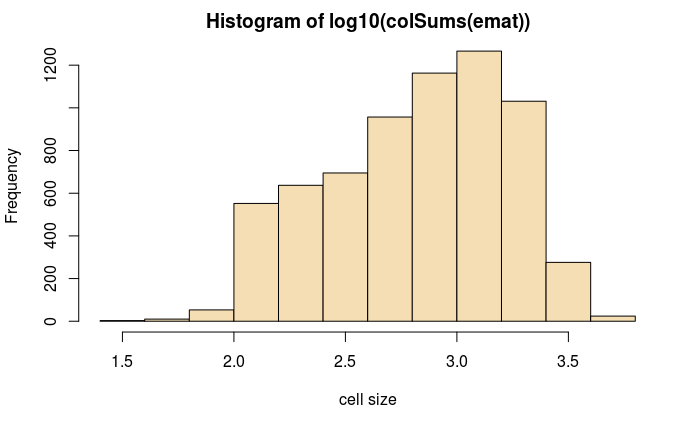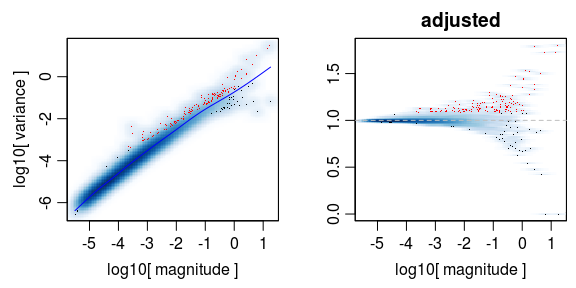## Molecule annotation

For inDrop experiments, the spliced/unspliced molecules can be annotated by:

1. Using dropEst output pipeline to produce a 10x-like bam file: `~/dropEst/build/dropest -m -F -L eiEIBA -o run1 -g cellranger/refdata-cellranger-mm10-1.2.0/genes/genes.gtf -c ~/dropEst/configs/indrop_v3.xml *.bam`

2. Using velocyto.py to annotated spliced and unspliced reads, writing out a standard loom file: `velocyto run -u Gene -o out -e SCG71 -m mm10_rmsk_srt.gtf -v SCG_71_tophat.filtered.sorted.bam UCSC/mm10/Annotation/Genes/genes.gtf`

(note that it is also possible to annotated spliced/unspliced reads with dropEst directly, using `-V` option: `~/dropEst/dropest -V -C 6000 -m -g ucsc_mm10_exons.gtf.gz -c ~/dropEst/configs/indrop_v3.xml *.aligned.bam`)

Please see the following shell script for a full set of commands used to prepare this particular example.

The example below starts with a loom file produced by velocyto.py, uses pagoda2 to obtain cell clusters/embedding, and then estimate/visualize velocity.

``````library(velocyto.R)
``````
``Loading required package: Matrix``

Load loom matrices: (to download pre-calculated loom matrices use `wget http://pklab.med.harvard.edu/velocyto/mouseBM/SCG71.loom`)

``````ldat <- read.loom.matrices(url("http://pklab.med.harvard.edu/velocyto/mouseBM/SCG71.loom"))
``````
``Error: is.character(name) is not TRUE``

## Normalize and cluster cells using pagoda2

Using spliced expression matrix as input to pagoda2.

``````emat <- ldat\$spliced
hist(log10(colSums(emat)),col='wheat',xlab='cell size')````````````# this dataset has already been pre-filtered, but this is where one woudl do some filtering
emat <- emat[,colSums(emat)>=1e3]``````

## Pagoda2 processing

Pagoda2 is used to generate cell embedding, cell clustering, as well as a more accurate cell-cell distance matrix. You can alternatively generate those using other tools, such as Seurat2, etc.

``````library(pagoda2)
r <- Pagoda2\$new(emat,modelType='plain',trim=10,log.scale=T)``````
``2600 cells, 7301 genes; normalizing ... using plain model winsorizing ... log scale ... done.``
``r\$adjustVariance(plot=T,do.par=T,gam.k=10)``
``calculating variance fit ... using gam 137 overdispersed genes ... 137 persisting ... done.``Run basic analysis steps to generate cell embedding and clustering, visualize:

``r\$calculatePcaReduction(nPcs=100,n.odgenes=3e3,maxit=300)``
``running PCA using 3000 OD genes ..``
``Loading required package: irlba``
``.. done``
``r\$makeKnnGraph(k=30,type='PCA',center=T,distance='cosine');``
``````Loading required package: igraph

Attaching package: ‘igraph’

The following objects are masked from ‘package:stats’:

decompose, spectrum

The following object is masked from ‘package:base’:

union``````
``````creating space of type angular done
building index ... done
querying ... done``````
``````r\$getKnnClusters(method=multilevel.community,type='PCA',name='multilevel')
r\$getEmbedding(type='PCA',embeddingType='tSNE',perplexity=50,verbose=T)``````
``````calculating distance ... pearson ...running tSNE using 16 cores:
Read the 2600 x 2600 data matrix successfully!
OpenMP is working...
Using no_dims = 2, perplexity = 50.000000, and theta = 0.500000
Computing input similarities...
Building tree...
Done in 9.36 seconds (sparsity = 0.084143)!
Learning embedding...
Iteration 50: error is 73.294295 (50 iterations in 3.70 seconds)
Iteration 100: error is 65.648297 (50 iterations in 3.19 seconds)
Iteration 150: error is 65.151406 (50 iterations in 3.17 seconds)
Iteration 200: error is 65.090414 (50 iterations in 3.24 seconds)
Iteration 250: error is 65.075571 (50 iterations in 3.27 seconds)
Iteration 300: error is 1.814009 (50 iterations in 3.11 seconds)
Iteration 350: error is 1.699950 (50 iterations in 3.08 seconds)
Iteration 400: error is 1.660607 (50 iterations in 3.01 seconds)
Iteration 450: error is 1.644676 (50 iterations in 2.98 seconds)
Iteration 500: error is 1.638744 (50 iterations in 2.96 seconds)
Iteration 550: error is 1.633982 (50 iterations in 3.00 seconds)
Iteration 600: error is 1.629732 (50 iterations in 2.99 seconds)
Iteration 650: error is 1.628101 (50 iterations in 3.12 seconds)
Iteration 700: error is 1.625991 (50 iterations in 3.27 seconds)
Iteration 750: error is 1.624012 (50 iterations in 3.15 seconds)
Iteration 800: error is 1.622847 (50 iterations in 3.27 seconds)
Iteration 850: error is 1.621847 (50 iterations in 3.31 seconds)
Iteration 900: error is 1.620831 (50 iterations in 3.34 seconds)
Iteration 950: error is 1.619936 (50 iterations in 3.21 seconds)
Iteration 1000: error is 1.618511 (50 iterations in 3.16 seconds)
Fitting performed in 63.52 seconds.``````

Plot embedding, labeling clusters (left) and “Xist” expression (which separates the male and female )

``````par(mfrow=c(1,2))
r\$plotEmbedding(type='PCA',embeddingType='tSNE',show.legend=F,mark.clusters=T,min.group.size=10,shuffle.colors=F,mark.cluster.cex=1,alpha=0.3,main='cell clusters')
r\$plotEmbedding(type='PCA',embeddingType='tSNE',colors=r\$depth,main='depth')  ``````
``treating colors as a gradient with zlim: 1000.9 2939 ``